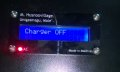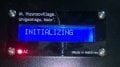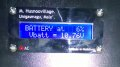# SLA Charger/Backup System

#### R!f@@

Joined Apr 2, 2009
9,861
It's Done.
Working exactly how I wanted.

#### JohnInTX

Joined Jun 26, 2012
4,712
Nothing succeeds like success!
Well done!

•R!f@@

#### MMcLaren

Joined Feb 14, 2010
859
Congrats!

•R!f@@ and JohnInTX

#### R!f@@

Joined Apr 2, 2009
9,861
Hey.

I rewrote whole code again to get familiar. This time TMR0 is only for the buzzer. And TMR1 is used at 5ms and TMR1 Derived timers at 125ms, 250ms and 1sec. Different times used like LCD refresh rate and all.

It's better than the one before I believe, added a battery testing function and some Leds too.

One more function is there to show the battery drain percentage. Basically it now shows at 10% intervals. Easy to do but I like to know how to go from 0 to 100% after checking ADC value at 1% increment.

Write now, 0% is 10.6V battery voltage and 100% is 12.6V.
I like to know how to show percentage vary from 0 to 100% when ADC changes like 700 to 1023. Any value below 700 is considered 0%.

What I did is really long. I know there is a better and way tht uses less code and some math
Thanks

#### JohnInTX

Joined Jun 26, 2012
4,712
@R!f@@
Its a simple slope-offset conversion.
The offset is 700 counts since 0% is 10.6V which reads 700 counts. So you subtract that offset from the ADC reading to locate the zero.

The span is 1023 - 700 counts giving 2V = 100% = 323 counts. That count range is mapped to the full scale value of 100 giving 3.23 counts per percent. Multiply by 100 to express the fraction as a percentage.
The resulting formula is then

It works in either integer or floating point arithmetic. In integer arithmetic, the result is an integer from 0 <= x <=100. I'd use integer arithmetic since the ranges are known in advance and scaling is convenient. Note where the *100 multiplier is. In integer arithmetic, you do it there to scale that numerator up before division. If you did it the school book way (divide then multiply by 100) you'd lose the fractional part in the integer division.

Have fun!

•R!f@@

#### R!f@@

Joined Apr 2, 2009
9,861
@JohnInTX
Thank you John.
Works like a charm.

This was simpler than I thought.

#### JohnInTX

Joined Jun 26, 2012
4,712
@JohnInTX
Thank you John.
Works like a charm.

This was simpler than I thought.
Cool! Glad it worked. What I described comes up pretty often when you have to scale one linear function (from a sensor etc) to another (readout etc). The trick of scaling an integer by some factor before division is useful in many applications as well.

#### R!f@@

Joined Apr 2, 2009
9,861It was after putting a new battery that I figured float charge was not needed. When Battery is bulk charged the open voltage is actually more than the float charge voltage. The picture with "FL-Charge was taken when it was switching from NL(Normal) to FL(Float) charge. so the displayed voltage is wrong. It is actually 13.65V. I had discard the float routine.
The battery drops to nominal voltage after a few minutes as a result the program skips the float mode. I guess it's OK.

All I need now is to see how actually it turns out in usage.

#### JohnInTX

Joined Jun 26, 2012
4,712
@R!f@@
That looks really good. Congratulations!
I'm glad you put 'Made in Maldives' on it, too. Nice to see homegrown talent in action!
Well done!

•R!f@@